# Factors, Primes and Prime Factorization

A factor of an integer n is any number that “goes into” n without remainder; n can be divided by it without a remainder.

For example, 6 is a factor of 12 because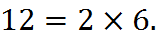Indeed, any multiplication can be thought of in the terms,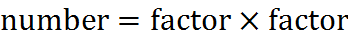Accordingly, the number 4 is not a factor of 13 because 4 cannot multiply any other whole number and come out with an even 13.

Every number has itself and 1 as factors. For example, 12 and 1 are both factors of 12. In some cases, a number’s only factors are itself and 1; such numbers are prime numbers, as we will discuss below.

Any number is divisible by its factors. Just as 12 is 2 times 6, as in our example above, 12 is divisible by 2 and by 6.

## Divisibility Rules

Divisibility rules are technically not required knowledge for the GMAT, but they are quite useful. A divisibility rule allows you determine, without actually performing the division, whether one number is divisible by another.

Here are some divisibility rules:

 DIVISOR N IS DIVISIBLE BY DIVISOR IF N… 2 is even 3 consists of digits that sum to a number divisible by 3 4 is even and remains even when divided by 2 5 has a final digit of 0 or 5 6 is divisible by both 2 and 3 (matches both rules above) 9 consists of digits that sum to a number divisible by 9 10 has a final digit of 0

For example, we can determine that the number 2,223,432,762 is divisible by 3 without performing long division, because 2 + 2 + 2 + 3 + 4 + 3 + 2 + 7 + 6 + 2 = 33, and 33 is divisible by 3. Similarly, that number is not divisible by 9, because 33 is not divisible by 9.

If you have fallen in love with these rules and want to learn as many as possible (that might sound odd, but I’ve seen it happen), there are many to learn, such as on the “Divisibility Rule” page on Wikipedia. However, you don’t really need any rules beyond the above, even for a top Quant score. That’s true in part because the most powerful technique to understand factors is to use prime numbers, as we’ll now discuss.

## Prime Numbers

An integer is called “prime” if its only factors are 1 and n. The prime numbers begin with 2: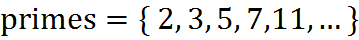Since “1 and n” are not two different numbers when 1 itself is n, this definition does not apply to the number 1 and 1 is not considered a prime number.

There are infinitely many primes. How soon the next prime number comes after a given prime number is generally not obvious.

Notice that 2 is the smallest prime number and the only even prime number. You will use this fact on GMAT questions.

## Identifying a Prime

On some GMAT questions, you will have to determine whether one or more numbers are prime. Here is the simplest method. The idea is that you try to show a number is not prime until you have run into a wall and the only possibility left is that it’s prime.

To determine whether a number n is prime:

1. Start with 2. You start with the number 2 and see whether 2 is a factor of n. If 2 is a factor, you are done: n is not prime.

2. Then 3. If the number 2 is not a factor of n, you try 3, using divisibility rules. If 3 is a factor, you are done; the number is not prime.

3. Continue up with primes… If the number 3 is not a factor, you try 5; then 7, 11, 13, 17, and the ascending primes (though you are probably done at 17).

4. …until you cross the “middle point.” You will know you are finished when you reach a “middle point.” There is a middle point close to the square root of n. After you pass it, you are just trying combinations of factors that you have already tried.

For example, let’s use this method to determine whether 117 is prime. We start with 2, but 2 is not a factor of 117, since 117 is odd. So we move on to 3. In fact, 3 is a factor of 117, since 1 + 1 + 7 = 9 and 9 is divisible by 3. Therefore, 117 is not a prime number.

Let’s try another example: 109. We start by trying 2. The number 109 is odd, so it’s not divisible by 2. Its digits sum to 1 + 0 + 9 = 10, and 10 is not divisible by 3, so 109 is not divisible by 3. We move up to 5. The number 109 does not end in 5 or 0, so it’s not divisible by 5. To see whether 7 is a factor, we can begin a long division; 7 goes into 109… 15 times, but with a remainder, so 7 is not a factor. The number 11 is not a factor because 11 times 10 is 110, which is one off from 109. And now we have crossed the middle point for 109, since the square root of 109 is between 10 and 11 (since 109 is between 100 and 121). Therefore, 109 is a prime number.

Why, when we are testing the factors in ascending order, do we have to try only the prime numbers? The reason is that, in testing the primes, we also test the non-primes. Take 6, for example. If we have already tried 2 and 3 as factors, we don’t need to bother trying 6, because 6 is simply 2 times 3, and if neither 2 nor 3 is a factor, than they certainly aren’t both factors, as would be required for 6 to be a factor.

## Prime Factorization

Every positive number can be factored into a product of primes. For example,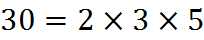where 2, 3 and 5 are prime. The factorization of a number into only primes is called its prime factorization. Every number that is not itself prime has exactly one prime factorization.

Every factorization of a number is either its prime factorization or an equivalent factorization involving one or more non-primes. The factorizations of 30 are listed below: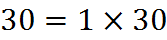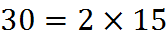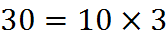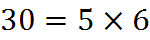What, then, is the prime factorization of 100?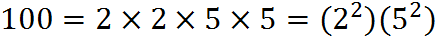We’ll now discuss how to find any number’s prime factorization.

## Using Factor Trees to Find a Prime Factorization

If you take a number and divide it into factors, and keep dividing its factors until you can divide no further, you will end up with the original number’s prime factorization. The successive divisions can be depicted as a “tree,” and the prime factorization of the original number will be the product of all the numbers left hanging at the end – the “leaves” of the tree.

For example, to find the prime factorization of 42, you might start by dividing by 2. That will give you this factor tree: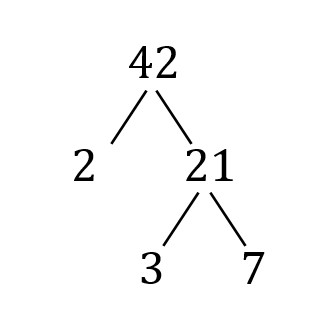The “leaves” of this tree are 2, 3, and 7, so the prime factorization of 42 is (2)(3)(7).

If you had started by dividing 42 into 6 and 7, you would have gotten a different tree, but the same factorization: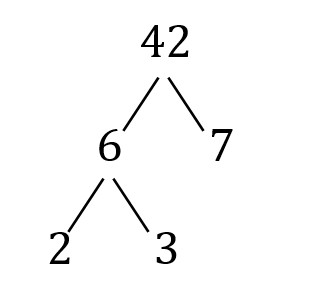Factor trees are quick and easy to sketch. Make use of them on your noteboard when doing GMAT questions. You want to avoid trying to do too much of a GMAT question in your head, leaving yourself open to preventable errors.

A factor tree gives you the prime factorization of a number, but it doesn’t directly give you all the factors of a number. We’ll now cover two ways to find all the factors of a number.

## How to Determine the Factors of a Number

Let’s discuss two ways to determine all the factors of some number n. It would be quite normal for a GMAT question to ask you to do this.

Testing Factors from 2 to the “Middle Point.” The first method, the simpler one, is a lot like the method above to determine whether a number is prime. You start with 2, attempting to divide into n as you go up, until you reach the “middle point” for that number. However, since you care about all factors, you try all integers, not just prime factors.

For example, given 42, you start with 2 and observe that 2 times 21 is 42. So 2 and 21 are factors, beyond the starting factors of 1 and 42. Next, 3 is a factor, as you can confirm by divisibility rules (4 + 2 = 6); 3 times 14 is 42. We come to 6 and 7, and then we are at the middle point, because after 6 and 7 come 7 and 6 (or, put another way, 6 squared is below 42 and 7 squared is above 42). Collecting them, we have 1, 2, 3, 6, 7, 14, 21, and 42. Notice they can be grouped in pairs around the middle point.

Forming Combinations of the Prime Factorization. The second method, which is more powerful but trickier, is to start by finding the prime factorization of n. In the case of 42, we have 42 = (2)(3)(7). Those three factors are like the “atoms” that make up the product 42. All possible factorizations of 42 must contain exactly those factors – no more, no less. So, if we exhaust the ways that 2, 3, and 7 can be divided into two numbers, we obtain all the factors of 42.

This method might be useful if you are asked (on a pretty difficult question) to determine how many factors a very large number has. On such a question, factor math meets the math of counting methods and combinations, discussed near the end of this book.

## Exercises

1. Is 4 a factor of 20?

Answer: Yes. The number 4 is a factor of 20 because 20 is 4 times 5.

2. Is 6 a factor of 20?

Answer: No. The number 6 is not a factor of 20 because 6 does not go into 20 without a remainder; it goes in three times with a remainder of 2.

3. Find all factorizations of 20. Which factorization of 20 is the prime factorization?

Answer: The factorizations of 20 are: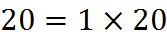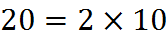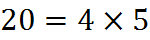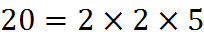This last factorization is the prime factorization of 20.

## Practice Questions

Sum of Primes:
http://www.gmatfree.com/sum-of-primes

Divisibility into Paperclip Piles:
http://www.gmatfree.com/divisibility-into-paperclip-piles

Maximum Divisors:
http://www.gmatfree.com/maximum-divisors

Welcome! You are encouraged to register with the site and login (for free). When you register, you support the site and your question history is saved.